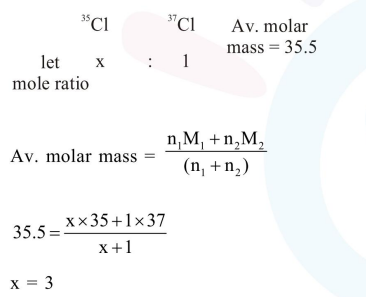# The average molar mass of chlorine is 35.5g

Question:

The average molar mass of chlorine is $35.5 \mathrm{~g}$ $\mathrm{mol}^{-1}$. The ratio of ${ }^{35} \mathrm{Cl}$ to ${ }^{37} \mathrm{Cl}$ in naturally occurring chlorine is close to :

1. $4: 1$

2. $1: 1$

3. $2: 1$

4. $3: 1$

Correct Option: , 4

Solution: